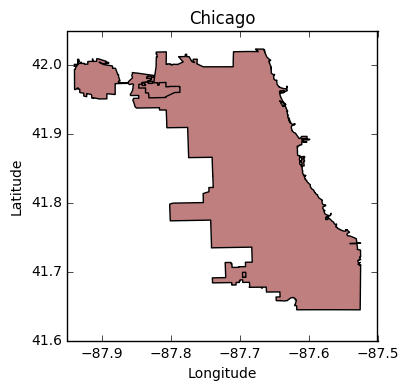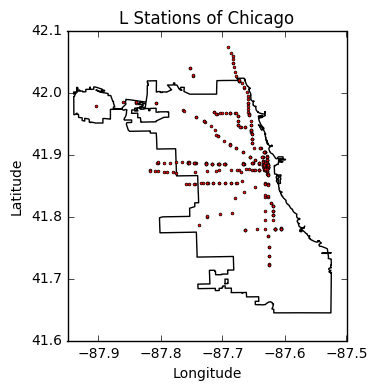# A Quick Intro To Interactive Visualizations With Bokeh¶

1. Introduction

2. GeoPandas

3. Bokeh

4. Conclusion

## Introduction ¶

The purpose of this blog post is to go over some of the basics of plotting with Bokeh. Bokeh is a Python library that generates interactive visualizations with ease and also can handle very large or streaming datasets. This is important because Matplotlib and Seaborn will often fail if the datasets one is working with becomes too large.

We will be using geospatial data with the end goal of making an interactive plot of the Chicago "L" stations. Therefore we will also make use of the GeoPandas library which extends the power of Pandas to geospatial data analysis.

We first cover how to work with GeoPandas and then dive into plotting with Bokeh. The source code for this blog post can be found here.

## GeoPandas ¶

GeoPandas extends the ease of working with the Pandas library for data analysis to geospatial analysis. It also has built-in plotting mechanisms that use the Matplotlib library. To start we include the following libraries:

In :
import geopandas as gpd
import matplotlib.pyplot as plt
% matplotlib inline


We next import the geojson files that contain the geometry of Chicago and also the "L" station entrances using the read_file() function from GeoPandas:

In :
Chi = gpd.read_file('chicago.geojson')


We can get a basic plot of outline of Chicago just by using the plot() function on the GeoPandas GeoDataFrame:

In :
Chi.plot()
plt.title('Chicago')
plt.xlabel('Longitude')
plt.ylabel('Latitude')

Out:
<matplotlib.text.Text at 0x10e5a1590>By default the different shapes/polygons in the plots will filled in with different colors. We can set the color of the city to be white by using the command,

Chi.plot(color = 'white')



We can also overlay the "L" stations with the above outline of Chicago. To do so we first set the city boundaries to be the "base map" with the command,

base_map = Chi.plot(color='white')



Then we plot the "L" stations on top of the base map by passing base_map to the plot function call as,

L_Stations.plot(ax=base_map, color='red')



The results can be seen below,

In :
base_map = Chi.plot(color='white')
L_Stations.plot(ax=base_map,color='red')
plt.title('L Stations of Chicago')
plt.xlabel('Longitude')
plt.ylabel('Latitude')

Out:
<matplotlib.text.Text at 0x1117becd0>But what if we want something more interactive? Something where we can zoom in or if we hover over a station we can get more information on it? For interactive plotting there are many options such as Carto which is easy to use and has a nice interface. However, you have to pay to use Carto. A free option is to use the D3.js library. But D3 has more of a learning curve and requires a lot of knowledge of JavaScript.

There is also somewhat inbetween the two called Bokeh.

## Bokeh ¶

Bokeh is free, is easy to use, doesn't require much JavaScript and gives you almost all of the capabilities of D3.js. It works on small, large and even streaming datasets which makes it perfect for data science and web analytics. The first thing we need to do to use Bokeh is to import the basic modules from library. Bokeh is written in a modular format and requires that each individual component be imported seperately. We read in the basic function modules below,

In :
from bokeh.io import output_file, output_notebook, show
from bokeh.plotting import figure, ColumnDataSource
output_notebook()


For the purposes of this introduction there are two outputing formats from Bokeh, one which writes the plot to a html file which is imported from output_file and one that directly embeds the html plot into the Jupyter notebook which is imported from output_notebook. We'll be working with output_notebook, which is why we have the declaration after all the imports,

output_notebook()



Now, let's get started with a simple non-interactive plot of Chicago. In order to accomplish this we have to write our GeoPandas DataFrame into format that Bokeh understands. Let's first take a look at the GeoPandas dataframe values,

In :
Chi.head()

Out:
geometry
0 (POLYGON ((-87.664207 42.021263, -87.664186 42...

We can see that it contains one column named geometry and contains one entry that is a polygon. A polygon is basically a list of points that are on the boundary of Chicago and have the format (Longitude Latitude). We need to extract the longitudes and latitudes into a collection of column-like lists that we can then feed into Bokeh.

We do this using the two functions below:

In :
def convert_GeoPandas_to_Bokeh_format(gdf):
"""
Function to convert a GeoPandas GeoDataFrame to a Bokeh
ColumnDataSource object.

:param: (GeoDataFrame) gdf: GeoPandas GeoDataFrame with polygon(s) under
the column name 'geometry.'

:return: ColumnDataSource for Bokeh.
"""
gdf_new = gdf.drop('geometry', axis=1).copy()
gdf_new['x'] = gdf.apply(getGeometryCoords,
geom='geometry',
coord_type='x',
shape_type='polygon',
axis=1)

gdf_new['y'] = gdf.apply(getGeometryCoords,
geom='geometry',
coord_type='y',
shape_type='polygon',
axis=1)

return ColumnDataSource(gdf_new)

def getGeometryCoords(row, geom, coord_type, shape_type):
"""
Returns the coordinates ('x' or 'y') of edges of a Polygon exterior.

:param: (GeoPandas Series) row : The row of each of the GeoPandas DataFrame.
:param: (str) geom : The column name.
:param: (str) coord_type : Whether it's 'x' or 'y' coordinate.
:param: (str) shape_type
"""

# Parse the exterior of the coordinate
if shape_type == 'polygon':
exterior = row[geom].geoms.exterior
if coord_type == 'x':
# Get the x coordinates of the exterior
return list( exterior.coords.xy )

elif coord_type == 'y':
# Get the y coordinates of the exterior
return list( exterior.coords.xy )

elif shape_type == 'point':
exterior = row[geom]

if coord_type == 'x':
# Get the x coordinates of the exterior
return  exterior.coords.xy

elif coord_type == 'y':
# Get the y coordinates of the exterior
return  exterior.coords.xy


The first function, convert_GeoPandas_to_Bokeh_format(), copies over the Pandas DataFrame into a new one. It then makes a new column in the DataFrame labeled 'x' which corresponds to the longitudes and 'y' which corresponds to the latitudes. Finally it returns the new DataFrame as a Bokeh data source called ColumnSourceData.

In the function convert_GeoPandas_to_Bokeh_format the longitudes and latitudes are extracted from the Polygon through the use of the function getGeometryCoords(). Inside getGeometryCoords() the data is broken into two cases:

- The source data is from Polygon. This is for the boundary of Chicago.

- The source data is from a lists of Points. These points will be the "L" stations.



We can now convert the Chicago boundary DataFrame into its corresponding Bokeh data source.

In :
Chi_Source = convert_GeoPandas_to_Bokeh_format(Chi)


Now we can do the actual plotting of the boundary of Chicago using the multi_line() function,

In :
p = figure(title="Chicago")
p.multi_line('x', 'y', source=Chi_Source, color="black", line_width=2)
show(p)


You can see that in order to plot with Bokeh we need to pass in the the fact that we are plotting 'x' and 'y' coorindates. These are also the name of the columns in the ColumnSourceData and correspond to the longitude and latitude values respectively.

Now say we want to build an interactive plot which shows all the "L" stations as points in Chicago and that if we hover over them with our mouse we get some additional information. In order to do this we need to import some more libraries from Bokeh,

In :
from bokeh.models import (
Range1d,
GeoJSONDataSource,
HoverTool,
LinearColorMapper,
GMapPlot, GMapOptions, ColumnDataSource,
Circle, DataRange1d, PanTool, WheelZoomTool, BoxSelectTool
)


We then need to convert all the points in L_Stations GeoPandas DataFrame into one that Bokeh can work with. We do this using the getGeometryCoords() function again. However, this time we show the effect it has on L_Station DataFrame before and after the function call. Before calling the getGeometryCoords() the DataFrame looks like,

In :
L_Stations.head()

Out:
agency geometry line name
0 CTA POINT (-87.669144 41.857849) Pink Line 18th
1 CTA POINT (-87.680632 41.829274) Orange Line 35th/Archer
2 CTA POINT (-87.62441 41.722729) Red Line 95th-Dan Ryan
3 CTA POINT (-87.625997 41.879715) Brown, Purple, Orange, Pink, Green Lines Adams/Wabash
4 CTA POINT (-87.718406 41.946604) Blue Line Addison
In :
L_Stations['x'] = L_Stations.apply(getGeometryCoords,
geom='geometry',
coord_type='x',
shape_type='point',
axis=1)

L_Stations['y'] = L_Stations.apply(getGeometryCoords,
geom='geometry',
coord_type='y',
shape_type='point',
axis=1)

L_Stations = L_Stations.drop(['geometry','agency'],axis=1)


And after the DataFrame looks like,

In :
L_Stations.head()

Out:
line name x y
0 Pink Line 18th -87.669144 41.857849
1 Orange Line 35th/Archer -87.680632 41.829274
2 Red Line 95th-Dan Ryan -87.624410 41.722729
3 Brown, Purple, Orange, Pink, Green Lines Adams/Wabash -87.625997 41.879715
4 Blue Line Addison -87.718406 41.946604

Now since we want to produce an interactive visualization we need to give Bokeh more information than just the latitude and longitudes of the "L" stations. We'll add the name of the station as well as the line it is on by creating a ColumnSourceData object from "scratch." We first make a dictionary of all the values and pass this dictionary as data when instantiate a ColumnSourceData object:

In :
L_Source = ColumnDataSource(data=dict(x=L_Stations['x'],
y=L_Stations['y'],
line=L_Stations['line'].values,
name=L_Stations['name'].values))


Now we write the function to overlay the "L" stations with the boundary of Chicago using Chi_Source and L_Source. We first set the tools we want our interactive plot to use,

In :
TOOLS = "pan,wheel_zoom,box_zoom,reset,hover,save"


Then instantiate our figure object to include those tools,

In :
Elevated = figure(title="Chicago \'L\' System",
tools=TOOLS,
x_axis_location=None,
y_axis_location=None)


We next plot the boundary of Chicago just as we did before,

In :
Elevated.multi_line('x',
'y',
source=Chi_Source,
color="black",
line_width=2)

Out:
GlyphRenderer(
id = '67a1f455-d2f6-4ede-82a8-34eaac45e243', …)

And plot the L stations using the circle() function. This adds a circle over all the longitudes and latitudes of the stations as well as passing the name and line information to the plotting routine as well.

In :
Elevated.circle('x',
'y',
source=L_Source,
size=4)

Out:
GlyphRenderer(
id = 'f31fdfe8-acb0-4a34-974c-02a0c126bb7f', …)

Here is where we add the interactive component to our visualization. We add the hover tool to our figure and set the "policy" for this hover tool to be that it follows the mouse. We do this through the command,

In :
hover = Elevated.select_one(HoverTool)
hover.point_policy = "follow_mouse"


Now we set the information to be displayed when one hovers over a "L" station by setting the tooltips to be an array of the information to be displayed:

In :
hover.tooltips = [
("Name", "@name"),
("Line.", "@line"),
("(Long, Lat)", "($x,$y)"),
]


Notice how each entry in the array is a tupple with the first component being the name of the variable and the second component being the variable value. We can now plot the interactive plot using the show() command:

In :
show(Elevated)


We can see that hovering over some stations produces multiple pop-ups. This is because the geojson file, cta_entrances.geojson, contains the entrances for each "L" stations and some stations have multiple entrances. You can observe this by zooming in on a station with multiple values in the pop-up and see the distinct entrances more clearly.

## Conclusion ¶

Bokeh is a powerful high performance Python visualization library that makes D3-like interactive web plotting easy. In this blogpost we went over how to make simple interactive plot using GeoPandas and Bokeh using the Chicago "L" station data as our example. There is definitely much more you can do with Bokeh, but that will have to wait for another day. I'll be updating this to add more cool features, so check back in the future!